The Australian Journal of Mathematical Analysis and Applications

 Home News Editors Volumes RGMIA Subscriptions Authors Contact

ISSN 1449-5910

You searched for agarwal
Total of 48 results found in site

8: Paper Source PDF document

Paper's Title:

On Oscillation of Second-Order Delay Dynamic Equations on Time Scales

Author(s):

S. H. Saker

Department of Mathematics, Faculty of Science,
Mansoura University, Mansoura, 35516,
Egypt.
shsaker@mans.edu.eg

Abstract:

Some new oscillation criteria for second-order linear delay dynamic equation on a time scale T are established. Our results improve the recent results for delay dynamic equations and in the special case when T=R, the results include the oscillation results established by Hille [1948, Trans. Amer. Math. Soc. 64 (1948), 234-252] and Erbe [Canad. Math. Bull. 16 (1973), 49-56.] for differential equations. When T=Z the results include and improve some oscillation criteria for difference equations. When T=hZ, h>0, T=qN and T=N2, i.e., for generalized second order delay difference equations our results are essentially new and can be applied on different types of time scales. An example is considered to illustrate the main results.

5: Paper Source PDF document

Paper's Title:

Oscillation and Boundedness of Solutions to First and Second Order Forced Dynamic Equations with Mixed Nonlinearities

Author(s):

Ravi P. Agarwal and Martin Bohner

Department of Mathematical Sciences, Florida Institute of Technology
Melbourne, FL 32901,
U.S.A.
bohner@mst.edu
URL:http://web.mst.edu/~bohner

Department of Economics and Finance, Missouri University of Science and Technology
Rolla, MO 65401,
U.S.A.
agarwal@fit.edu

Abstract:

Some oscillation and boundedness criteria for solutions to certain first and second order forced dynamic equations with mixed nonlinearities are established. The main tool in the proofs is an inequality due to Hardy, Littlewood and Pólya. The obtained results can be applied to differential equations, difference equations and q-difference equations. The results are illustrated with numerous examples.

4: Paper Source PDF document

Paper's Title:

Existence of Non-spurious Solutions to Discrete Boundary Value Problems

Author(s):

Irena Rachunkova and Christopher C. Tisdell

Department of Mathematics
Palacky University
771 46 Olomouc, Czech Republic.
rachunko@risc.upol.cz
URL: http://phoenix.inf.upol.cz/~rachunekl/mathair/matha-en.htm

School of Mathematics
The University of New South Wales
Sydney 2052, Australia.
cct@unsw.edu.au
URL: http://www.maths.unsw.edu.au/~cct

Abstract:

This paper investigates discrete boundary value problems (BVPs) involving second-order difference equations and two-point boundary conditions. General theorems guaranteeing the existence and uniqueness of solutions to the discrete BVP are established. The methods involve a sufficient growth condition to yield an a priori bound on solutions to a certain family of discrete BVPs. The em a priori bounds on solutions to the discrete BVP do not depend on the step-size and thus there are no ``spurious'' solutions. It is shown that solutions of the discrete BVP will converge to solutions of ordinary differential equations.

3: Paper Source PDF document

Paper's Title:

Inequalities of Gamma Function Appearing in Generalizing Probability Sampling Design

Author(s):

Department of Mathematics,
Yarmouk University,
Irbid 21163,
Jordan.

Department of Statistics,
Yarmouk University,
Irbid 21163,
Jordan.

Abstract:

In this paper, we investigate the complete monotonicity of some functions involving gamma function. Using the monotonic properties of these functions, we derived some inequalities involving gamma and beta functions. Such inequalities can be used to generalize different probability distribution functions. Also, they can be used to generalize some statistical designs, e.g., the probability proportional to the size without replacement design.

2: Paper Source PDF document

Paper's Title:

Positive Solutions of Evolution Operator Equations

Author(s):

Department of Applied Mathematics,
Babes-Bolyai University,
Cluj, Romania

Abstract:

Existence and localization results are derived from Krasnoselskii’s compressionexpansion fixed point theorem in cones, for operator equations in spaces of continuous functions from a compact real interval to an abstract space. The main idea, first used in , is to handle two equivalent operator forms of the equation, one of fixed point type giving the operator to which Krasnoselskii’s theorem applies and an other one of coincidence type which is used to localize a positive solution in a shell. An application is presented for a boundary value problem associated to a fourth order partial differential equation on a rectangular domain.

2: Paper Source PDF document

Paper's Title:

Positive Solutions for Systems of Three-point Nonlinear Boundary Value Problems

Author(s):

J. Henderson and S. K. Ntouyas

Department of Mathematics, Baylor University
Waco, Texas
76798-7328 USA.
Johnny_Henderson@baylor.edu
URL: http://www3.baylor.edu/~Johnny_Henderson

Department of Mathematics, University of Ioannina
451 10 Ioannina,
Greece.
sntouyas@cc.uoi.gr
URL: http://www.math.uoi.gr/~sntouyas

Abstract:

Values of λ are determined for which there exist positive solutions of the system of three-point boundary value problems, u''(t)+ λa(t)f(v(t))=0, v''(t)+λb(t)g(u(t))=0, for 0 < t <1, and satisfying, u(0) = 0, u(1)=α u(η), v(0) = 0, v(1)=α v(η). A Guo-Krasnosel'skii fixed point theorem is applied.

2: Paper Source PDF document

Paper's Title:

Some New Nonlinear Integro-Differential Inequalities of Gronwall-Bellman-Pachpatte Type

Author(s):

A. ABDELDAIM

Department of Mathematics and Computer Sciences,
Faculty of Science,
Port Said University, Port Said,
EGYPT.

Department of Mathematics,
Faculty of Science and Humanities,
SAUDI ARABIA.

E-mail: ahassen@su.edu.sa
URL: http://faculty.ksu.edu.sa/DRABDELDAIM/Pages/Home.aspx

Abstract:

In this paper we establish some new nonlinear integro-differential inequalities of Gronwall-Bellman-Pachpatte type for function of one independent variable. The purpose of this paper is to extend certain results which proved by Pachpatte in [On some fundamental integrodifferential and integral inequalities, An. Sti. Univ. Al. I. Cuza, Iasi, Vol.23 (1977), 77-86]. The inequalities obtained here can be used in the theory of some new classes of nonlinear integro-differential equations. Some applications are also given to illustrate the usefulness of our results.

2: Paper Source PDF document

Paper's Title:

Strong Convergence Theorem for a Common Fixed Point of an Infinite Family of J-nonexpansive Maps with Applications

Author(s):

Charlse Ejike Chidume, Otubo Emmanuel Ezzaka and Chinedu Godwin Ezea

African University of Science and Technology,
Abuja,
Nigeria.
E-mail: cchidume@aust.edu.ng

Ebonyi State University,
Abakaliki,
Nigeria.
E-mail: mrzzaka@yahoo.com

Nnamdi Azikiwe University,
Awka,
Nigeria.
E-mail: chinedu.ezea@gmail.com

Abstract:

Let E be a uniformly convex and uniformly smooth real Banach space with dual space E*. Let {Ti}i=1 be a family of J-nonexpansive maps, where, for each i,~Ti maps E to 2E*. A new class of maps, J-nonexpansive maps from E to E*, an analogue of nonexpansive self maps of E, is introduced. Assuming that the set of common J-fixed points of {Ti}i=1 is nonempty, an iterative scheme is constructed and proved to converge strongly to a point x* in n=1FJTi. This result is then applied, in the case that E is a real Hilbert space to obtain a strong convergence theorem for approximation of a common fixed point for an infinite family of nonexpansive maps, assuming existences. The theorem obtained is compared with some important results in the literature. Finally, the technique of proof is also of independent interest.

2: Paper Source PDF document

Paper's Title:

Strong Convergence Theorems for a Common Zero of an Infinite Family of Gamma-Inverse Strongly Monotone Maps with Applications

Author(s):

Charles Ejike Chidume, Ogonnaya Michael Romanus, and Ukamaka Victoria Nnyaba

African University of Science and Technology, Abuja,
Nigeria.
E-mail: cchidume@aust.edu.ng
E-mail: romanusogonnaya@gmail.com
E-mail: nnyabavictoriau@gmail.com

Abstract:

Let E be a uniformly convex and uniformly smooth real Banach space with dual space E* and let Ak:EE*, k=1, 2, 3 , ...
be a family of inverse strongly monotone maps such that k=1 Ak-1(0)≠∅.
A new iterative algorithm is constructed and proved to converge strongly to a common zero of the family.
As a consequence of this result, a strong convergence theorem for approximating a common J-fixed point for an infinite family of
gamma-strictly J-pseudocontractive maps is proved. These results are new and improve recent results obtained for these classes of nonlinear maps.
Furthermore, the technique of proof is of independent interest.

2: Paper Source PDF document

Paper's Title:

Convergence and Stability Results for New Three Step Iteration Process in Modular Spaces

Author(s):

Naresh Kumar and Renu Chugh

Department of Mathematics,
M.D. University,
Rohtak-124001, Haryana,
India.
E-mail: nks280@gmail.com
E-mail: chugh.r1@gmail.com

Abstract:

The aim of this paper is to introduce a new iteration process (5) for ρ-contraction mappings in Modular spaces. We obtain some analytical proof for convergence and stability of our iteration process (5). We show that our iteration process (5) gives faster convergence results than the leading AK iteration process (4) for contraction mappings. Moreover, a numerical example (using the Matlab Software) is presented to compare the rate of convergence for existing iteration processes with our new iteration process (5).

1: Paper Source PDF document

Paper's Title:

Positive Solution For Discrete Three-Point Boundary Value Problems

Author(s):

Wing-Sum Cheung And Jingli Ren

Department of Mathematics,
The University of Hong Kong,
Pokfulam, Hong Kong
wscheung@hku.hk

Institute of Systems Science,
Beijing 100080, P.R. China
renjl@mx.amss.ac.cn

Abstract:

This paper is concerned with the existence of positive solution to the discrete three-point boundary value problem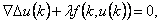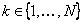,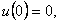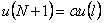where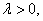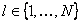, and f is allowed to change sign. By constructing available operators, we shall apply the method of lower solution and the method of topology degree to obtain positive solution of the above problem for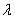on a suitable interval. The associated Green’s function is first given.

1: Paper Source PDF document

Paper's Title:

Oscillations of First Order Linear Delay Difference Equations

Author(s):

G. E. Chatzarakis and I. P. Stavroulakis

Department of Mathematics, University of Ioannina,
451 10, Greece
ipstav@cc.uoi.gr

Abstract:

Consider the first order linear delay difference equation of the form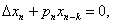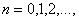where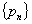is a sequence of nonnegative real numbers, k is a positive integer and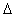denotes the forward difference operator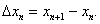New oscillation criteria are established when the well-known oscillation conditions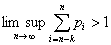and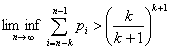are not satisfied. The results obtained essentially improve known results in the literature.

1: Paper Source PDF document

Paper's Title:

Boundary Value Problems for Fractional Diffusion-Wave equation

Author(s):

Varsha Daftardar-Gejji and Hossein Jafari

Department of Mathematics, University of Pune,
Ganeshkhind, Pune - 411007,
INDIA.
vsgejji@math.unipune.ernet.in
jafari_h@math.com

Abstract:

Non homogeneous fractional diffusion-wave equation has been solved under linear/nonlinear boundary conditions. As the order of time derivative changes from 0 to 2, the process changes from slow diffusion to classical diffusion to mixed diffusion-wave behaviour.
Numerical examples presented here confirm this inference. Orthogonality of eigenfunctions in case of fractional Stürm-Liouville problem has been established

1: Paper Source PDF document

Paper's Title:

Some Properties of the Solution of a Second Order Elliptic Abstract Differential Equation

Author(s):

A. Aibeche and K. Laidoune

Mathematics Department, Faculty of Sciences, University Ferhat Abbas, Setif,
Route de Scipion, 19000, Setif,
Algeria
aibeche@univ-setif.dz

Abstract:

In this paper we study a class of non regular boundary value problems for elliptic differential-operator equation of second order with an operator in boundary conditions. We give conditions which guarantee the coerciveness of the solution of the considered problem, the completeness of system of root vectors in Banach-valued functions spaces and we establish the Abel basis property of this system in Hilbert spaces. Finally, we apply this abstract results to a partial differential equation in cylindrical domain.

1: Paper Source PDF document

Paper's Title:

On a Method of Proving the Hyers-Ulam Stability of Functional Equations on Restricted Domains

Author(s):

Janusz Brzdęk

Department of Mathematics
Pedagogical University Podchor
ąźych 2,
30-084 Krak
ów,
Poland
jbrzdek@ap.krakow.pl

Abstract:

We show that generalizations of some (classical) results on the Hyers-Ulam stability of functional equations, in several variables, can be very easily derived from a simple result on stability of a functional equation in single variable

1: Paper Source PDF document

Paper's Title:

Common Fixed Point Results for Banach Operator Pairs and Applications to Best Approximation

Author(s):

Hemant Kumar Nashine

Department of Mathematics,
Disha Institute of Management and Technology,
Satya Vihar, Vidhansabha - Chandrakhuri Marg (Baloda Bazar Road), Mandir Hasaud,
Raipur - 492101(Chhattisgarh), India.

hemantnashine@rediffmail.com
nashine_09@rediffmail.com

Abstract:

The common fixed point results for Banach operator pair with generalized nonexpansive mappings in q-normed space have been obtained in the present work. As application, some more general best approximation results have also been determined without the assumption of linearity or affinity of mappings. These results unify and generalize various existing known results with the aid of more general class of noncommuting mappings.

1: Paper Source PDF document

Paper's Title:

Bounds for Two Mappings Associated to the Hermite-Hadamard Inequality

Author(s):

S. S. Dragomir1,2 and I. Gomm1

1Mathematics, School of Engineering & Science,
Victoria University,
PO Box 14428, Melbourne City, MC 8001,
Australia.

2School of Computational & Applied Mathematics,
University of the Witwatersrand,
Private Bag 3, Johannesburg 2050,
South Africa.

sever.dragomir@vu.edu.au
ian.gomm@vu.edu.au
URL: http://rgmia.org/dragomir

Abstract:

Some inequalities concerning two mappings associated to the celebrated Hermite-Hadamard integral inequality for convex function with applications for special means are given.

1: Paper Source PDF document

Paper's Title:

On Opial's Inequality for Functions of n-Independent Variables

Author(s):

S. A. A. El-Marouf and S. A. AL-Oufi

Department of Mathematics,
Faculty of Science,
Minoufiya University,
Shebin El-Koom,
Egypt

Department of Mathematics,
Faculty of Science, Taibah University,
Kingdom of Saudia Arabia

Abstract:

In this paper, we introduce Opial inequalities for functions of n-independent variables. Also, we discuss some different forms of Opial inequality containing functions of n independent variables and their partial derivatives with respect to independent variables.

1: Paper Source PDF document

Paper's Title:

Further Bounds for Two Mappings Related to the Hermite-Hadamard Inequality

Author(s):

S. S. Dragomir1,2 and I. Gomm1

1Mathematics, School of Engineering & Science,
Victoria University,
PO Box 14428, Melbourne City, MC 8001,
Australia.

2School of Computational & Applied Mathematics,
University of the Witwatersrand,
Private Bag 3, Johannesburg 2050,
South Africa.

sever.dragomir@vu.edu.au
ian.gomm@vu.edu.au
URL: http://rgmia.org/dragomir

Abstract:

Some new results concerning two mappings associated to the celebrated Hermite-Hadamard integral inequality for twice differentiable functions with applications for special means are given.

1: Paper Source PDF document

Paper's Title:

Some Applications of Fejér's Inequality for Convex Functions (I)

Author(s):

S.S. Dragomir1,2 and I. Gomm1

1Mathematics, School of Engineering & Science
Victoria University, PO Box 14428
Melbourne City, MC 8001,
Australia.

sever.dragomir@vu.edu.au

URL: http://rgmia.org/dragomir

2School of Computational & Applied Mathematics,
University of the Witwatersrand,
Private Bag 3, Johannesburg 2050,
South Africa.

Abstract:

Some applications of Fejér's inequality for convex functions are explored. Upper and lower bounds for the weighted integral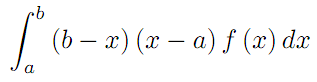under various assumptions for f with applications to the trapezoidal quadrature rule are given. Some inequalities for special means are also provided

1: Paper Source PDF document

Paper's Title:

Some Operator Order Inequalities for Continuous Functions of Selfadjoint Operators in Hilbert Spaces

Author(s):

S. S. Dragomir1,2 and Charles E. M. Pearce3

1Mathematics, School of Engineering & Science,
Victoria University,
PO Box 14428, Melbourne City, MC 8001,
Australia.

2School of Computational & Applied Mathematics,
University of the Witwatersrand,
Private Bag 3, Johannesburg 2050,
South Africa.

sever.dragomir@vu.edu.au
URL: http://rgmia.org/dragomir

3School of Mathematical Sciences,
Australia

Abstract:

Various bounds in the operator order for the following operator transform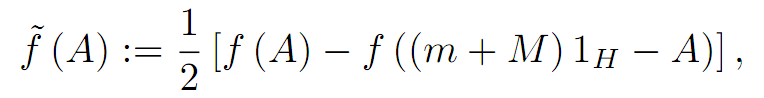where A is a selfadjoint operator in the Hilbert space H with the spectrum
Sp( A) ⊆ [ m,M] and f:[m,M] ->  C is a continuous function on [m,M]  are given. Applications for the power and logarithmic functions are provided as well.

1: Paper Source PDF document

Paper's Title:

Inequalities for the Area Balance of Functions of Bounded Variation

Author(s):

Sever S. Dragomir1,2

1Mathematics, School of Engineering & Science
Victoria University, PO Box 14428
Melbourne City, MC 8001,
Australia
E-mail: sever.dragomir@vu.edu.au

2DST-NRF Centre of Excellence in the Mathematical and Statistical Sciences,
School of Computer Science & Applied Mathematics,
University of the Witwatersrand,
Private Bag 3, Johannesburg 2050,
South Africa
URL: http://rgmia.org/dragomir

Abstract:

We introduce the area balance function associated to a Lebesgue integrable function f:[a,b] C by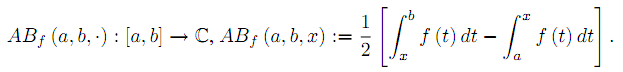Several sharp bounds for functions of bounded variation are provided. Applications for Lipschitzian and convex functions are also given.

1: Paper Source PDF document

Paper's Title:

Ostrowski Type Inequalities for Lebesgue Integral: a Survey of Recent Results

Author(s):

Sever S. Dragomir1,2

1Mathematics, School of Engineering & Science
Victoria University, PO Box 14428
Melbourne City, MC 8001,
Australia
E-mail: sever.dragomir@vu.edu.au

2DST-NRF Centre of Excellence in the Mathematical and Statistical Sciences,
School of Computer Science & Applied Mathematics,
University of the Witwatersrand,
Private Bag 3, Johannesburg 2050,
South Africa
URL: http://rgmia.org/dragomir

Abstract:

The main aim of this survey is to present recent results concerning Ostrowski type inequalities for the Lebesgue integral of various classes of complex and real-valued functions. The survey is intended for use by both researchers in various fields of Classical and Modern Analysis and Mathematical Inequalities and their Applications, domains which have grown exponentially in the last decade, as well as by postgraduate students and scientists applying inequalities in their specific areas.

1: Paper Source PDF document

Paper's Title:

Fractional class of analytic functions Defined Using q-Differential Operator

Author(s):

K . R. Karthikeyan, Musthafa Ibrahim and S. Srinivasan

Department of Mathematics and Statistics,
Caledonian College of Engineering, Muscat,
Sultanate of Oman.
E-mail: kr_karthikeyan1979@yahoo.com

College of Engineering,
University of Buraimi, Al Buraimi,
Sultanate of Oman.
E-mail: musthafa.ibrahim@gmail.com

Department of Mathematics, Presidency College (Autonomous),
India.

Abstract:

We define a q-differential fractional operator, which generalizes Salagean and Ruscheweyh differential operators. We introduce and study a new class of analytic functions involving q-differential fractional operator. We also determine the necessary and sufficient conditions for functions to be in the class. Further, we obtain the coefficient estimates, extreme points, growth and distortion bounds.

1: Paper Source PDF document

Paper's Title:

Some Inequalities of the Hermite-Hadamard Type for k-Fractional Conformable Integrals

Author(s):

C.-J. Huang, G. Rahman, K. S. Nisar, A. Ghaffar and F. Qi

Department of Mathematics, Ganzhou Teachers College,
Ganzhou 341000, Jiangxi,
China.
E-mail: hcj73jx@126.com , huangcj1973@qq.com

Department of Mathematics, Shaheed Benazir Bhutto University,
Sheringal, Upper Dir, Khyber Pakhtoonkhwa,
Pakistan.
E-mail: gauhar55uom@gmail.com

Department of Mathematics, College of Arts and Science at Wadi Aldawaser, 11991,
Prince Sattam Bin Abdulaziz University, Riyadh Region,
Kingdom of Saudi Arabia.
E-mail: n.sooppy@psau.edu.sa, ksnisar1@gmail.com

Department of Mathematical Science, Balochistan University of Information Technology,
Engineering and Management Sciences, Quetta,
Pakistan.
E-mail: abdulghaffar.jaffar@gmail.com

School of Mathematical Sciences, Tianjin Polytechnic University,
Tianjin 300387,
China; Institute of Mathematics,
Henan Polytechnic University, Jiaozuo 454010, Henan,
China.
E-mail: qifeng618@gmail.com, qifeng618@qq.com

Abstract:

In the paper, the authors deal with generalized k-fractional conformable integrals, establish some inequalities of the Hermite-Hadamard type for generalized k-fractional conformable integrals for convex functions, and generalize known inequalities of the Hermite-Hadamard type for conformable fractional integrals.

1: Paper Source PDF document

Paper's Title:

Author(s):

F. U. Ogbuisi

School of Mathematics, Statistics and Computer Science,
University of KwaZulu-Natal, Durban,
South Africa.

Department of Mathematics,
University of Nigeria, Nsukka,
Nigeria.
E-mail: ferdinard.ogbuisi@unn.edu.ng fudochukwu@yahoo.com

Abstract:

In this paper, we propose and analyze a type of subgradient extragradient algorithm for the approximation of a solution of variational inequality problem which is also a common fixed point of an infinite family of relatively nonexpansive mappings in 2-uniformly convex Banach spaces which are uniformly smooth. By using the generalized projection operator, we prove a strong convergence theorem which does not require the prior knowledge of the Lipschitz constant of cost operator. We further applied our result to constrained convex minimization problem, convex feasibility problem and infinite family of equilibrium problems. Our results improve and complement related results in 2-uniformly convex and uniformly smooth Banach spaces and Hilbert spaces.

Search and serve lasted 1 second(s).